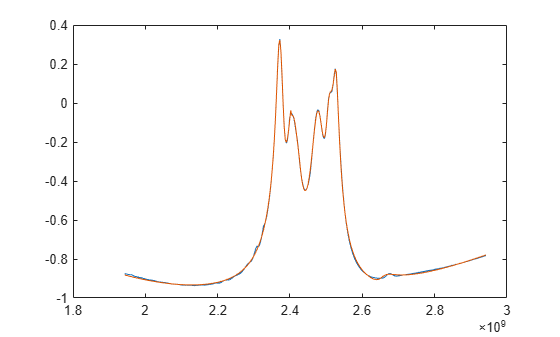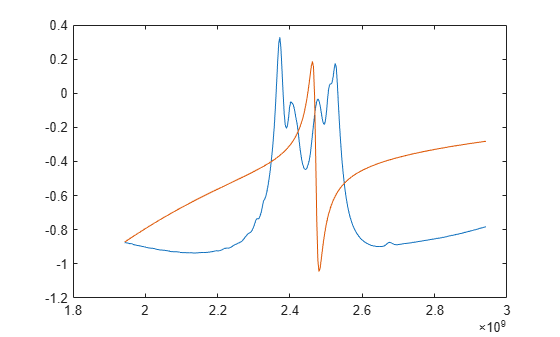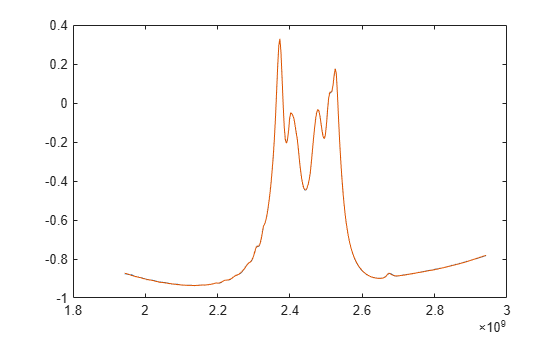# Using Rational Object to Fit S-Parameters

This example shows how to use the rational object to create a rational fit to S-parameter data, and the various properties and methods that are included in the rational object.

### Create Rational Object

Read in the `sparameters`, and create the rational object from them. The rational function automatically fits all entries of the S-parameter matrices.

`S = sparameters('sawfilter.s2p')`
```S = sparameters: S-parameters object NumPorts: 2 Frequencies: [334x1 double] Parameters: [2x2x334 double] Impedance: 50 rfparam(obj,i,j) returns S-parameter Sij ```
`r = rational(S)`
```r = rational with properties: NumPorts: 2 NumPoles: 24 Poles: [24x1 double] Residues: [2x2x24 double] DirectTerm: [2x2 double] ErrDB: -40.9658 ```

With the default settings on this example, the rational function achieves an accuracy of about -26 dB, using 30 poles. By construction, the rational object is causal, with a non-zero direct term.

### Compare Fit with Original Data

Generate the frequency response from the rational object, and compare one of the entries with the original data.

```resp = freqresp(r, S.Frequencies); plot(S.Frequencies, real(rfparam(S, 1, 1)), ... S.Frequencies, real(squeeze(resp(1,1,:))))```### Limit Number of Poles

Redo the fit, limiting the number of poles to a maximum of 5. The rational object may use fewer poles than specified. Notice that the quality of the fit is degraded as opposed to the original 30-pole fit.

`r5 = rational(S, 'MaxPoles', 5)`
```r5 = rational with properties: NumPorts: 2 NumPoles: 4 Poles: [4x1 double] Residues: [2x2x4 double] DirectTerm: [2x2 double] ErrDB: -1.7376 ```
```resp5 = freqresp(r5, S.Frequencies); plot(S.Frequencies, real(rfparam(S, 1, 1)), ... S.Frequencies, real(squeeze(resp5(1,1,:))))```### Tighten Target Accuracy

Redo the fit, asking for a tighter tolerance (-60 dB), Notice that the fit is significantly improved, particularly in the stopbands of the SAW filter.

`rgood = rational(S, -60)`
```rgood = rational with properties: NumPorts: 2 NumPoles: 188 Poles: [188x1 double] Residues: [2x2x188 double] DirectTerm: [2x2 double] ErrDB: -53.6395 ```
```respgood = freqresp(rgood, S.Frequencies); plot(S.Frequencies, real(rfparam(S, 1, 1)), ... S.Frequencies, real(squeeze(respgood(1,1,:))))```